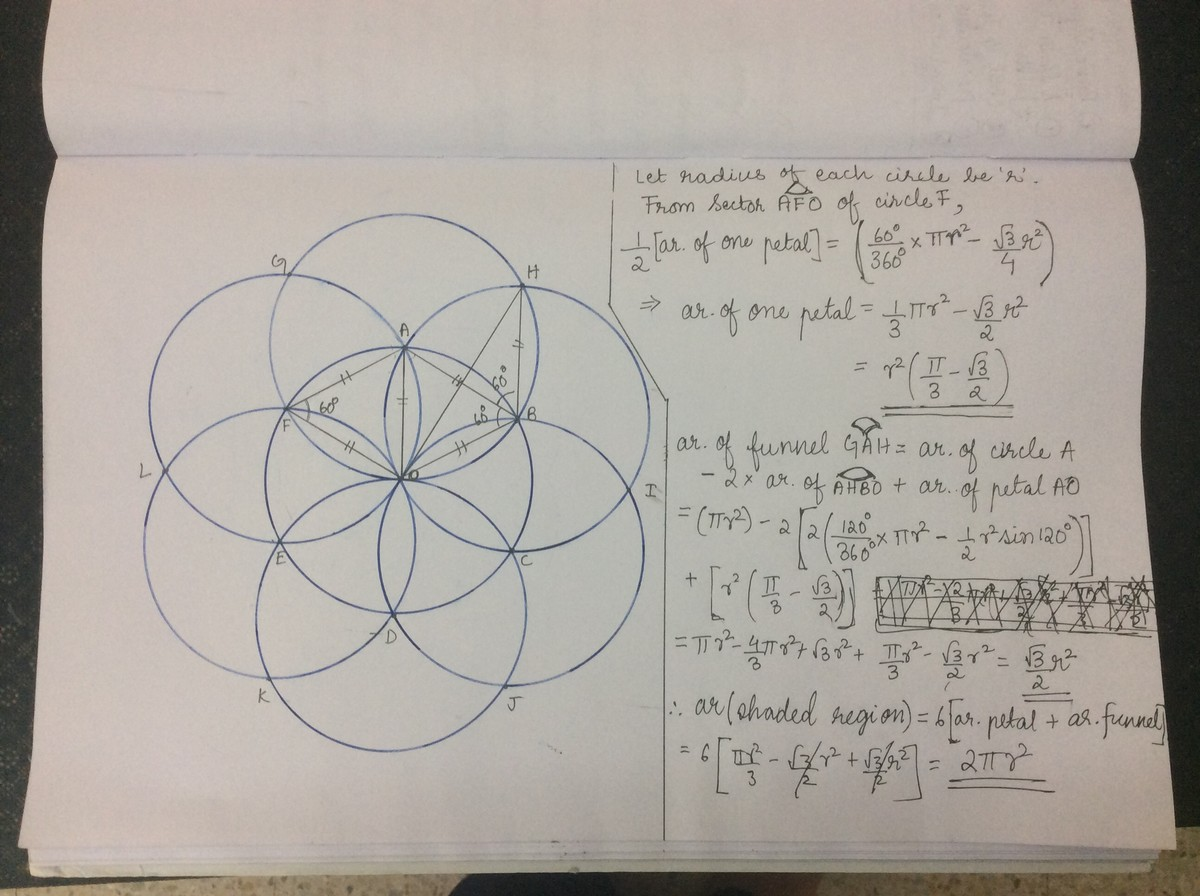# Seven circles, each with radius 1, overlap to form the composite figure shown below. Find the area of shaded region?

0 votes
651 views

Seven circles, each with radius 1, overlap to form the composite figure shown below. Find the area of shaded region?posted Nov 10, 2017
Share this puzzle

## 1 Answer

0 votes

### Explanation:

We can clearly see 6 "petals" (inner) and 6 "funnels" (outer). Now,So the area is 2πr^2 = 2πanswer Dec 28, 2017

Similar Puzzles
+1 vote

7 non-overlapping unit circles are inscribed inside a large circle as shown below. Find the area of the shaded region?+1 vote

Two congruent circles of radius 5 cm are drawn in such a way that one is 1 cm below the other . Find the area of red coloured region?+1 vote

Find the area of the blue shaded region in this 14x7 rectangle, with two semicircles of radius 7 drawn.0 votes

Two circles with the same radius overlap each other. Their circumferences cross each other's center. How much of the area of either circle in percentage is the overlapped area?+3 votes

A circle has a radius of 12 units and its center is at one vertex of a square. The square has a side of 12 units. Find the area of the shaded region?